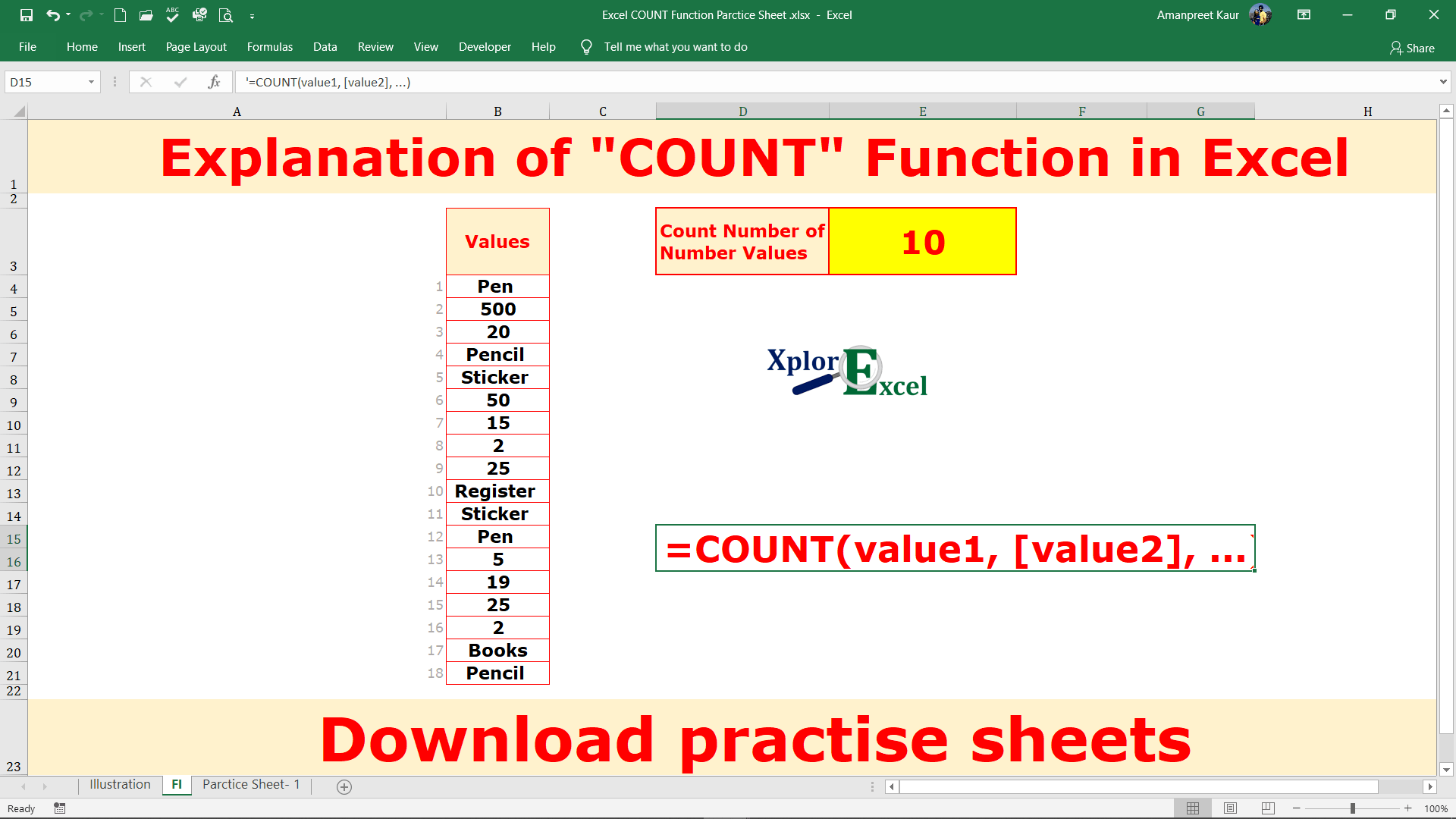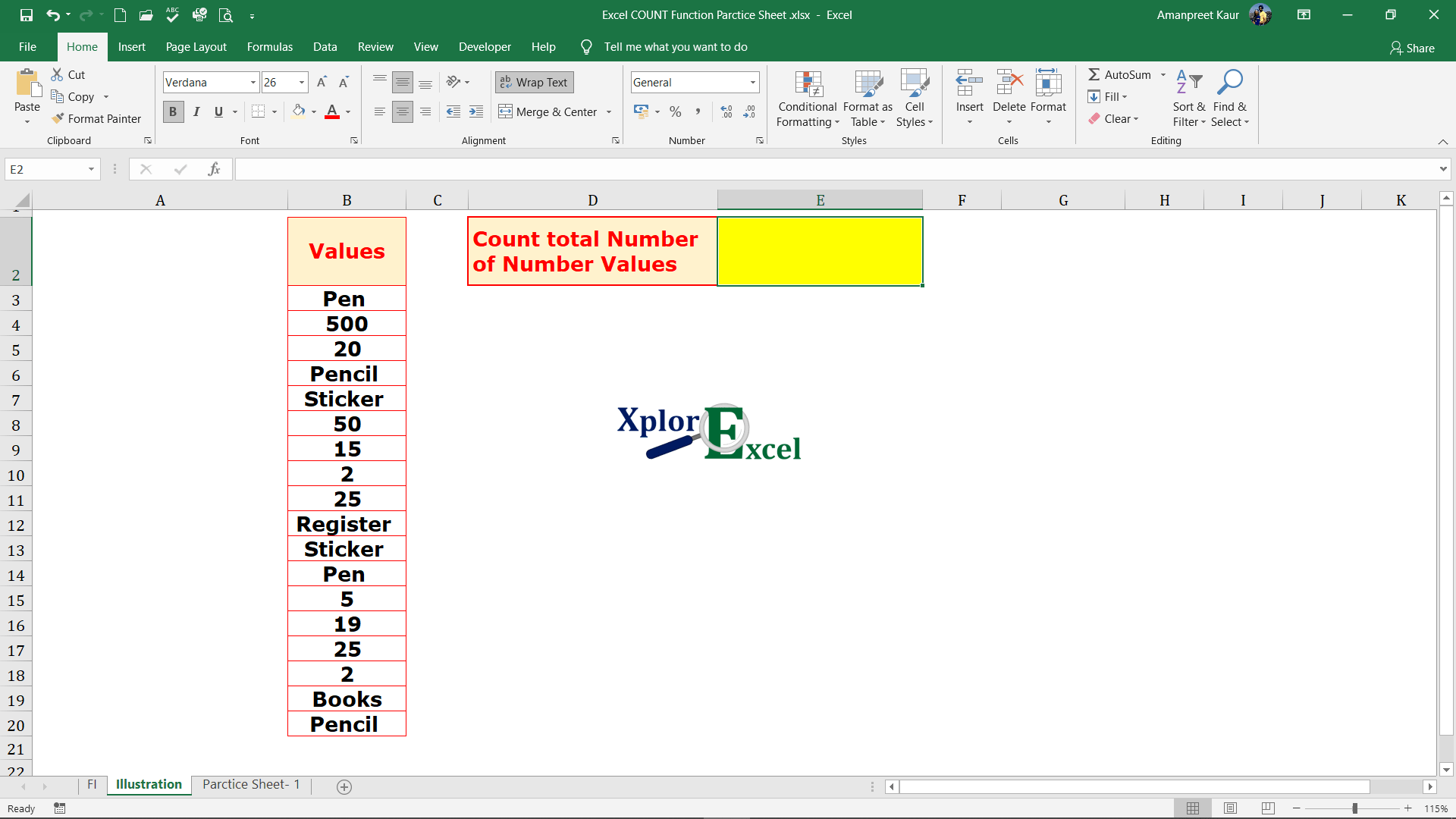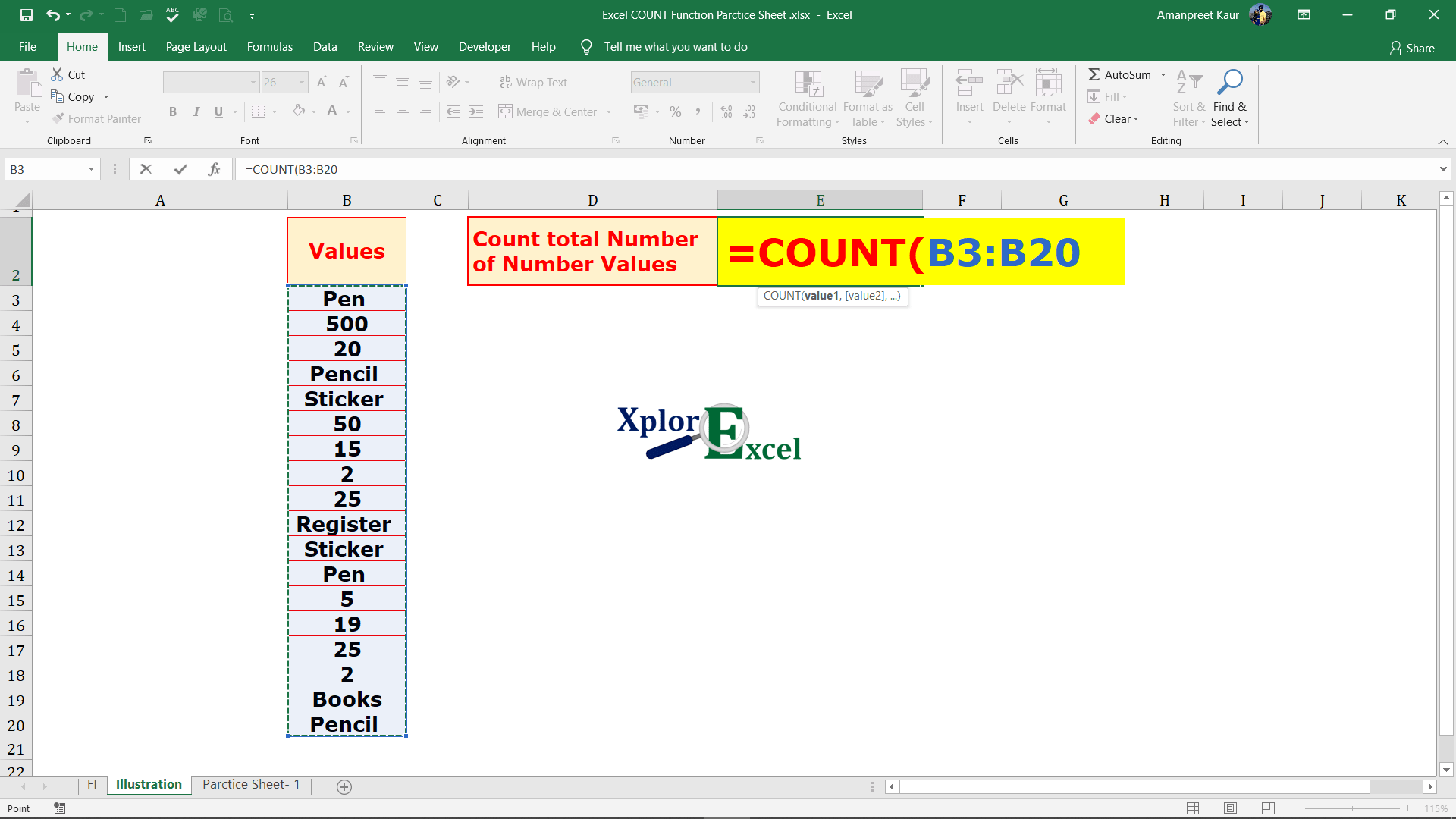## COUNT Function in Excel: –

The COUNT Function in Excel is used, where we need to count the number of numbers in the selected range or cell reference. It can count together with the numerical values in the multiple selected ranges or cell references.

• With the help of the COUNT Function, we can get the total number of the number values in the selected array, range, or cells.
• It can use further with other excel functions.
• Error-values or text values that cannot be recognized into numbers are not counted by this function.
• The logical values TRUE and FALSE are also not counted.
• Empty cells and text are also not counted.

### Explanation of Function:-

Now, We will explain the Arguments of the Function.

=COUNT(value1, [value2], …)

value1: – Value 1 is represented the selected range of the cells from which the function has to count the number of numbers values.

[value2]: – Value 2 is represented the second selected range of the cells from which the function has to count the number of numbers values. Because we can use multiple selections. If you have only a single row or column to select then you don’t need to enter the second value.

### Example of Function: –

I will show you, how to apply the function.

From the following table get the total number values in the values column.Solution:-

We will Apply the COUNT function and get the result. This is shown in the following steps: –

Step No. 1:-

We will write the “=COUNT( ” in the column of the result.Step No. 2

Now select the range of cells on which you want to apply the function.Step No. 3: –

Now, end the function with brackets then press enter and get the result: –Thanks

Also, Check out the following function of excel: –

### One Comment

1.#### zortilonrel

May 2, 2021

Howdy! I could have sworn I’ve been to this blog before but after checking through some of the post I realized it’s new to me. Anyhow, I’m definitely glad I found it and I’ll be bookmarking and checking back often!